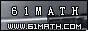Today is . WELCOME: huohai3983/44663  Home | Reg Login | All user | setHomePage | BookmarkHome | Math Test Online | Addition | Subtraction | Multiplication | Division | Time | Worksheet(JPG/PDF) | CAI | Flash Games |Recommendation articleArticle searchHome--maths--Second Grade--Mentally Subtracting Two-Digit Numbers

Mentally Subtracting Two-Digit Numbers

Mentally Subtracting Two-Digit Numbers

How to mentally subtract two two-digit numbers.

• Subtract 35 from 84.
• First subtract the two tens' place digits (8 - 3 = 5)
• Notice that the bottom ones' digit is larger than the top ones' digit. Decrease the answer for the tens' place by one (5 - 1 = 4) and increase the top ones' place value by 10 (4 + 10 = 14).
• Next subtract the two ones' place values (14 - 5 = 9)
• Combine the tens' and ones' place differences to make 49.
• Subtract 62 from 94.
• First subtract the two tens' place digits (9 - 6 = 3)
• Next subtract the two ones' place digits (4 - 2 = 2)
• Combine the tens' and ones' place differences to give the answer of 32

#### Find the Difference between the two Numbers.

 -     =

2006-12-15 22:44:53
Viewed 2990 times CloseGreatmathsites